# Essbase - Dynamic Calculations

When you design the overall database Essbase - Calculations, you may want to define a member as a Dynamic Calc member. When you Essbase - Tag a member as Dynamic Calc, Essbase calculates the Essbase - Combinations/Intersections of that member when you retrieve the data, instead of precalculating the member combinations during the regular database calculation.

Dynamic calculations shorten regular database calculation time but may increase retrieval time for dynamically calculated data values.

## Example

In the figure below, the TBC Measures dimension contains several members tagged as Dynamic Calc :

• Profit,
• Margin,
• Total Expenses,
• Margin %,
• and Profit %.

When an overall database calculation is performed, the Dynamic Calc members and their corresponding Essbase - Formulas are not calculated. These members are calculated when a user requests them.

## Storage

Essbase does not store the calculated values, it recalculates the values for any subsequent retrieval. However, you can choose to store dynamically calculated values after the first retrieval.

## When to calculate data values dynamically

To decide when to calculate data values dynamically, consider your priorities in the following areas:

• Optimum regular calculation time (batch calculation)
• Low disk space usage
• Reduced database restructure time
• Speedy data retrieval for users
• Reduced backup time

## Documentation / Reference

Discover More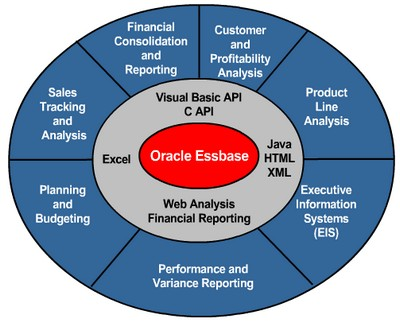Essbase - Calculations

Essbase includes powerful calculation features for demanding analytic requirements. A rich library of functions makes it easy to define advanced and sophisticated business logic and relationships. Essbase...Essbase - Consolidation Storage Properties

Data storage properties define where and when consolidations are stored. For example, by default, members are tagged as store data. Essbase sums the values of store data members and stores the result...Loading data is the process of adding data values to an database from a data source. If the data source is not perfectly formatted, you need a data load rules files to load the data values. You can load...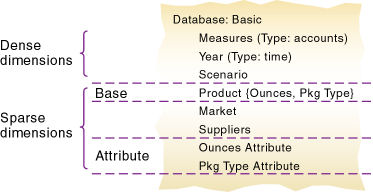Essbase - Designing an Outline to Optimize Query or Calculations Performance

The relative locations of dimensions in an outline can affect performance times : either or retrieval . Indeed, although they contain the same dimensions, the outline examples below : for Optimized...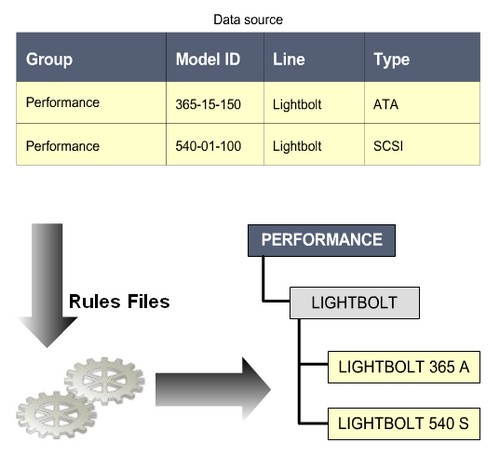Essbase - Dimension build rules files

When you create dimension with thousands of member or with many alternate hierarchy, you want to automate the process to add the member. You use Dimension build rules files to map dimension and member...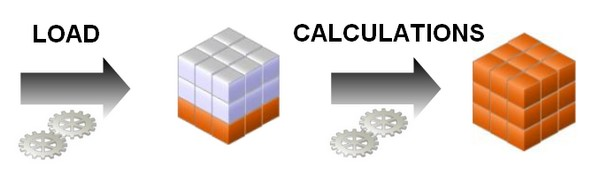Essbase - The OLAP Design Cycle (to create an optimized database)

A contains two types of values : the values that you enter or load (input data) and the values that are calculated from the input data (calculated data) Then a typical conforms to the following...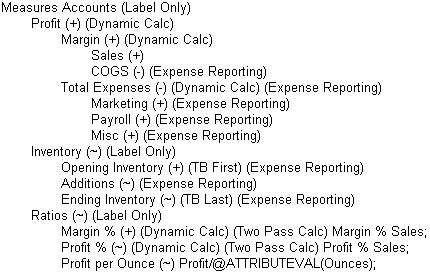Essbase - Two-Pass Calculations

This label indicates that some member must be calculated twice to produce the desired value. The two-pass property works only : on members of the dimension tagged as account and on members tagged...